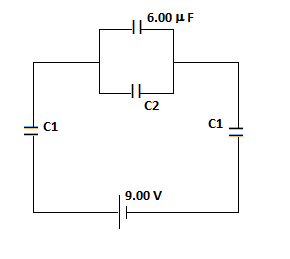# Consider the following. (Let C_1 = 35.80 \space \mu F and C_2 = 29.80 \space \mu F.) (a) Find...

## Question:

Consider the following. (Let {eq}C_1 = 35.80 \space \mu F {/eq} and {eq}C_2 = 29.80 \space \mu F {/eq}.)

(a) Find the equivalent capacitance of the capacitors in the figure.

(b) Find the charge on each capacitor.

(c) Find the potential difference across each capacitor.## Calculating Charge and Voltage:

When capacitors are connected in series, the charge on them is the same but the voltage may be different. When connected in parallel, the charge may be different but the voltage across them is the same.

#### Part (a)

To determine the equivalent capacitance, {eq}C_e {/eq} of the circuit, let's write the configuration of the capacitors and then...

Become a Study.com member to unlock this answer! Create your accountSeries & Parallel Capacitors

from UPSEE Paper 1: Study Guide & Test Prep

Chapter 11 / Lesson 9
7.3K

Support